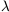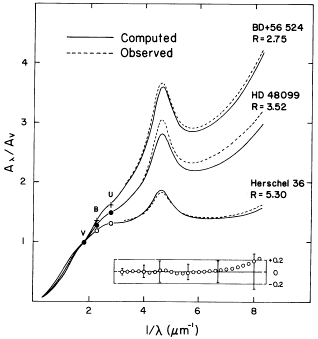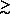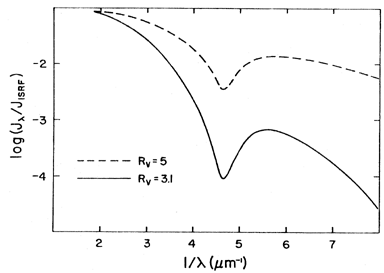Annu. Rev. Astron. Astrophys. 1990. 28: 37-70 Copyright © 1990 by Annual Reviews. All rights reserved

### 2. INTERSTELLAR EXTINCTION

2.1 Continuous Extinction

Each line of sight has its own ``extinction law'', or variation of extinction with wavelength, usually expressed by A() / A(V) in this article. This means of expressing the extinction law is not unique; it has been common practice to use instead the ratios of two colors, E (- V) / E (B - V), where E (- V) = A() - A(V). The use of A(V) as the reference extinction is arbitrary, and it might be preferable to use instead a longer wavelength, such as the J bandpass (1.25 µm), because the extinction law would then be virtually independent of line of sight (see Section 2.1.3). The information content of the extinction law is independent of how the law is expressed, but certain relations (see Section 2.1.1) become more obvious when A() / A(V) is used in place of E (- V) / E (B - V).

2.1.1. OPTICAL/ULTRAVIOLET EXTINCTION OF DIFFUSE AND OUTER-CLOUD DUST There have been several studies of the spatial distribution of extinction in the optical part of the spectrum (e.g., 51, 101, 122) that might be useful in estimating the amount of extinction in a particular direction, but this review concentrates instead upon the form of the extinction law and the physical nature of dust. Ardeberg and Virdefors (5) discuss the optical extinction law, with references.

Many authors have utilized the International Ultraviolet Explorer (IUE) to make detailed studies of the UV extinction law of diffuse dust and outer-cloud dust. There are considerable differences among the various lines of sight. Cardelli, Clayton, and Mathis [19, 20, (hereinafter CCM89)] have used the UV observations of Fitzpatrick and Massa [54 (hereinafter FM86), 55, 109], with optical/NIR observations of the same stars, to explore the relationships between various extinction laws over the entire available interval of wavelengths. These observations were spread over the sky and included both diffuse dust and lines of sight to the Ophiuchus, Orion, and other molecular clouds, as well as H II regions. CCM89 used the optical total-to-selective extinction ratio, RV = A(V) / E (B - V), as a parameter. (RV is determined by etrapolating NIR extinction to infinite wavelength.) Figure 1 shows the observed extinction laws of many lines of sight, plotted against RV-1, for several values ofranging from the red to almost the limit of the IUE spacecraft (1200 Å). There are fairly tight linear relationships between A() / A(V) and RV-1 in each case, including the UV wavelengths.Figure 1. The observations of A() / A(V) plotted against RV-1, where RV = A(V) / E(B - V) (from CCM89). A12 refers to 1200 Å, A22 to 2175 Å, A28 to 2800 Å, and A70 to 7000 Å (the standard R filter). The observational values of black dots are from Fitzpatrick and Massa (53). The regularity of the observations and the scatter about the mean relationship are also shown.

The value of RV depends upon the environment along the line of sight. A direction through low-density ISM usually has a rather low value of RV (about 3.1). Lines of sight penetrating into a dense cloud, such as the Ophiucus or Taurus molecular clouds, usually show 4 < RV < 6. However, it is not possible to estimate RV quantitatively from the environment of a line of sight; for example, the star VI Cyg 12 lies behind a dense cloud of dust, but has an RV of 3.1 (78), a value appropriate for the diffuse ISM. As a further example, RV3.0-3.5 in parts of the Taurus cloud (159).

CCM89 fitted the slopes of the various A() / A(V) - RV-1 relations, examples of which are shown in Figure 1, by an analytic formula that represents the mean extinction law as a function of RV. The expression is not reproduced here. Figure 2 (from CCM89) shows the mean extinction law for three values of RV as calculated from the formula. Also shown are actual observations with the same values of RV. The central curve is about as discrepant as actual observations are from the mean relationship. The dispersion of individual extinction laws around that mean law is shown in Figure 1 (from the spread in the individual observed points) and in the panel in Figure 2 (as error bars giving the standard deviation). The lowest set of curves plotted in Figure 2 are for Herschel 36, an exciting star of the H II region M8 and considered to have very ``peculiar'' extinction. Rather, Herschel 36 has a rather peculiar value of RV (5.3), but a ``normal'' extinction law for that value of RV. Note, however, that there are real deviations from the mean extinction law for any particular value of RV. These deviations are especially large at 1200 Å, where the standard deviation of A() / A(V) from the mean extinction law is about 0.3. Extreme deviations might be found in thefuture. Deviations will provide valuable information regarding the processes which modify the grains.Figure 2. Three cases of a mean extinction law. Solid lines are obtained by fitting the slopes of the A() / A(V) - RV-1 relationship (Figure 1) by an analytic formula (CCM89), and dashed lines are actual extinction laws of stars (FM86) with the appropriate values of RV. The error bars in the lower panel show the standard deviations of the observations of the entire sample (54) from the extinction law obtained from the formula. The open circles in the panel show the deviations from the ``standard'' mean extinction law (149) for the value RV = 3.2, appropriate for the diffuse ISM.

The ``mean'' extinction laws of Savage and Mathis (144) and Seaton (149) are commonly used to correct observations for the presence of dust. Both laws are reproduced closely if RV = 3.2 is substituted into the RV-dependent mean extinction law given in CCM89. The panel in Figure 2 shows the deviations of the CCM89 mean extinction law, with RV = 3.2 [from Seaton (149)]. It is not surprising that mean extinction laws correspond to an RV slightly higher than 3.1; some lines of sight used in the averaging penetrate fairly dense regions.

Figure 1 shows that there is a continuous change between properties of diffuse dust, with RV3.1, and outer-cloud dust, with large values of RV. The twod esignations merely contrast one end of the obseRVed range of RV with the other.

The differences of the extinctions between diffuse dust and outer-cloud dust strongly affect any predictions of physical conditions inside of clouds. Figure 2 shows tha outer-cloud extinction laws, i.e. those with R4, rise much less steeply at shorter wavelengths than diffuse dust. Consequently, interstellar radiation incident upon a cloud can penetrate the cloud much more easily than would be predicted from the Seaton or Savage-Mathis extinction laws. Figure 3 shows the mean radiation intensity at the center of a cloud with a radial extinction of A(V) = 5 magnitudes (a typical value) computed using RV = 3.1, and also with a typical outer-cloud dust value of RV = 5. The difference in the predicted mean intensities has large implications for the physical processes in the cloud.Figure 3. The mean intensity of radiation at the center of a cloud with a radial extinction of A(V) = 5 magnitudes and no internal sources, expressed in terms of the mean intensity of the incident interstellar radiation field. Two values of RV [= A(V) / E(B - V)] are shown: one corresponding to the mean value for diffuse dust (RV = 3.1), and one for a typical observed value for lines of sight penetrating clouds (RV = 5). The use of a mean extinction law corresponding to diffuse dust for predicting the radiation field within clouds leads to a gross error in the predicted radiation field within the cloud.

The fact that the extinction law depends so regularly on RV suggests that the processes which modify the sizes and/or the compositions of grains must operate on all sizes simultaneously and quite efficiently. One could imagine that the small and large grains would be modified independently along various lines of sight , but such is not the case. The consequences of the regularity of the various extinction laws are considered in Section 7.